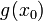# Taylor series operator commutes with composition

Suppose$x_0$ is a real number. Suppose$g$ is a function defined on a subset of the reals that is infinitely differentiable at$x_0$. Suppose$f$ is a function defined on a subset of the reals that is infinitely differentiable at$g(x_0)$. Then, the composite of two functions$f \circ g$ is infinitely differentiable at$x_0$, and its Taylor series can be computed formally by composing the Taylor series for$f$ at$g(x_0)$ with the Taylor series for$g$ at$x_0$. Formally, what this means is that we write down the Taylor series for$f$ at$g(x_0)$, then plug in for$x$ the entire expression for the Taylor series of$g$, then simplify.